My Life 时光荏苒，岁月如梭

# ruby 流程控制

2018-09-20
wsdjeg

Ruby 提供了其他现代语言中很常见的条件结构。在这里，我们将解释所有的条件语句和 Ruby 中可用的修饰符。

## if…else 语句

``````if conditional [then]
code...
[elsif conditional [then]
code...]...
[else
code...]
end
``````

if 表达式用于条件执行。值 false 和 nil 为假，其他值都为真。请注意，Ruby 使用 elsif，不是使用 else if 和 elif。 如果 conditional 为真，则执行 code。如果 conditional 不为真，则执行 else 子句中指定的 code。 通常我们省略保留字 then 。若想在一行内写出完整的 if 式，则必须以 then 隔开条件式和程式区块。如下所示:

``````if a == 4 then a = 7 end
``````

``````#!/usr/bin/ruby
# -*- coding: UTF-8 -*-

x=1
if x > 2
puts "x 大于 2"
elsif x <= 2 and x!=0
puts "x 是 1"
else
puts "无法得知 x 的值"
end
``````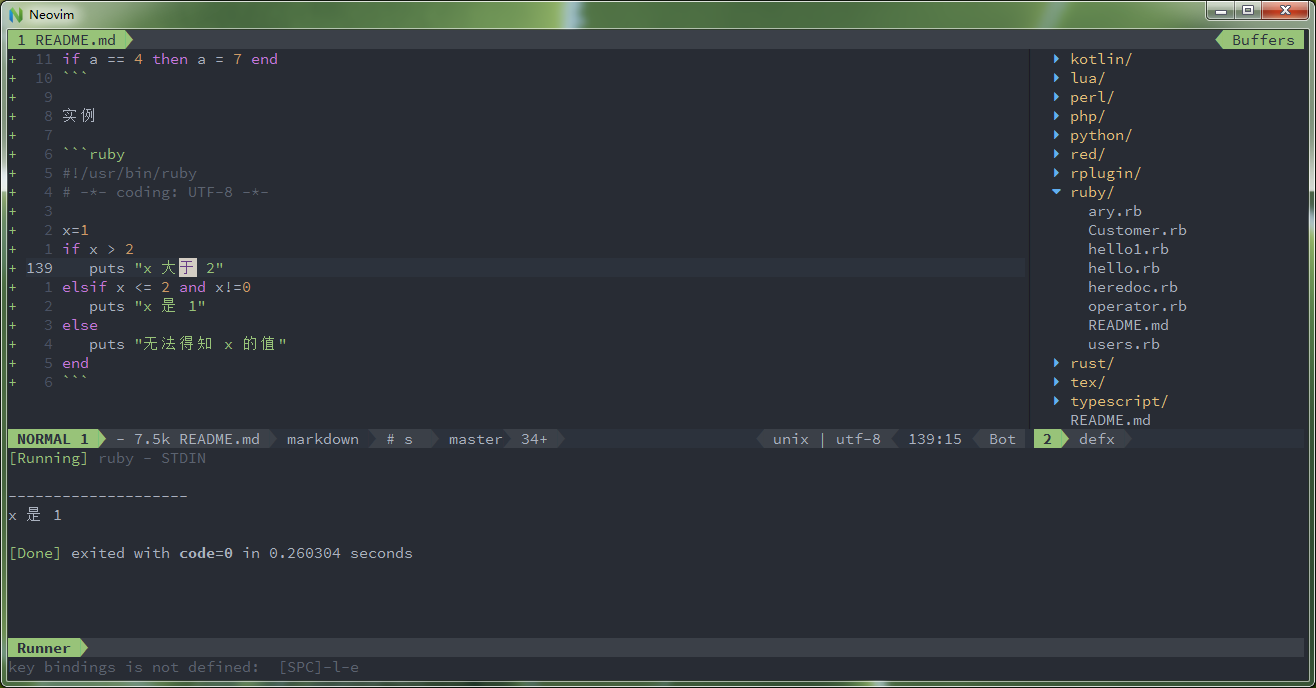## if 修饰符

``````code if condition
``````

``````#!/usr/bin/ruby

\$debug=1
puts "debug\n" if \$debug
``````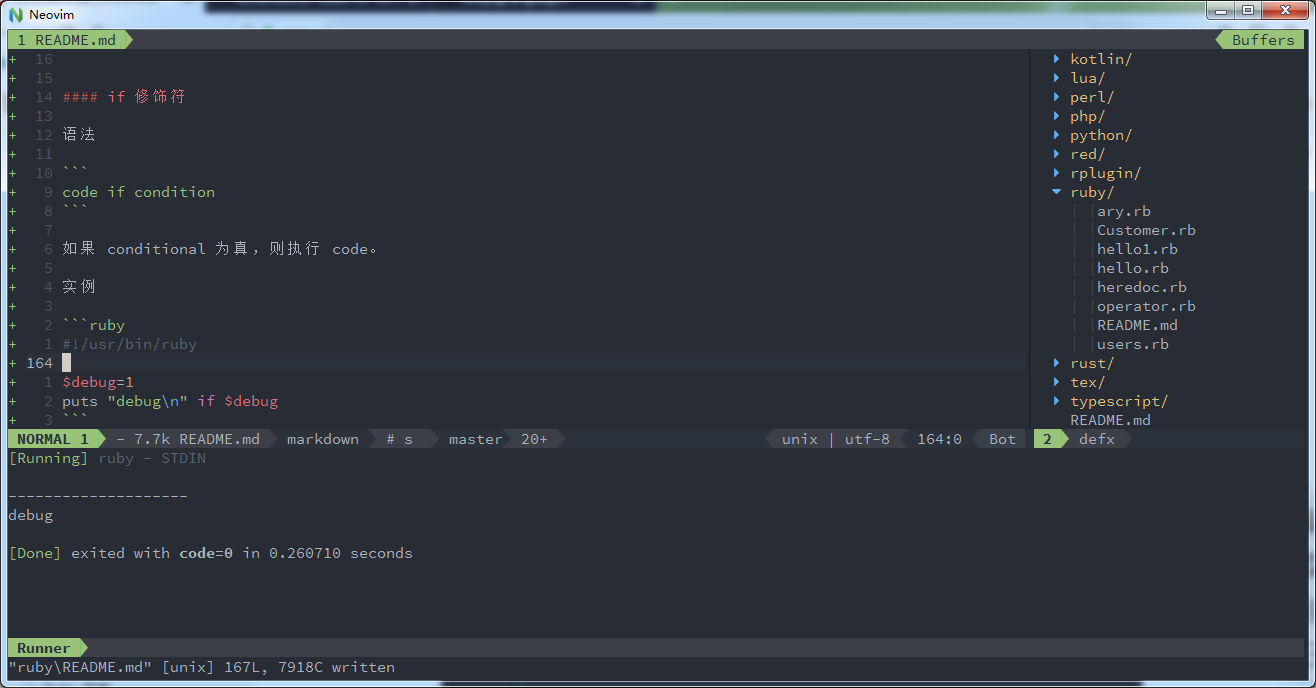## unless 语句

``````unless conditional [then]
code
[else
code ]
end
``````

unless 式和 if 式作用相反，即如果 conditional 为假，则执行 code。如果 conditional 为真，则执行 else 子句中指定的 code。

``````#!/usr/bin/ruby

x=1
unless x>2
puts "x 小于 2"
else
puts "x 大于 2"
end
``````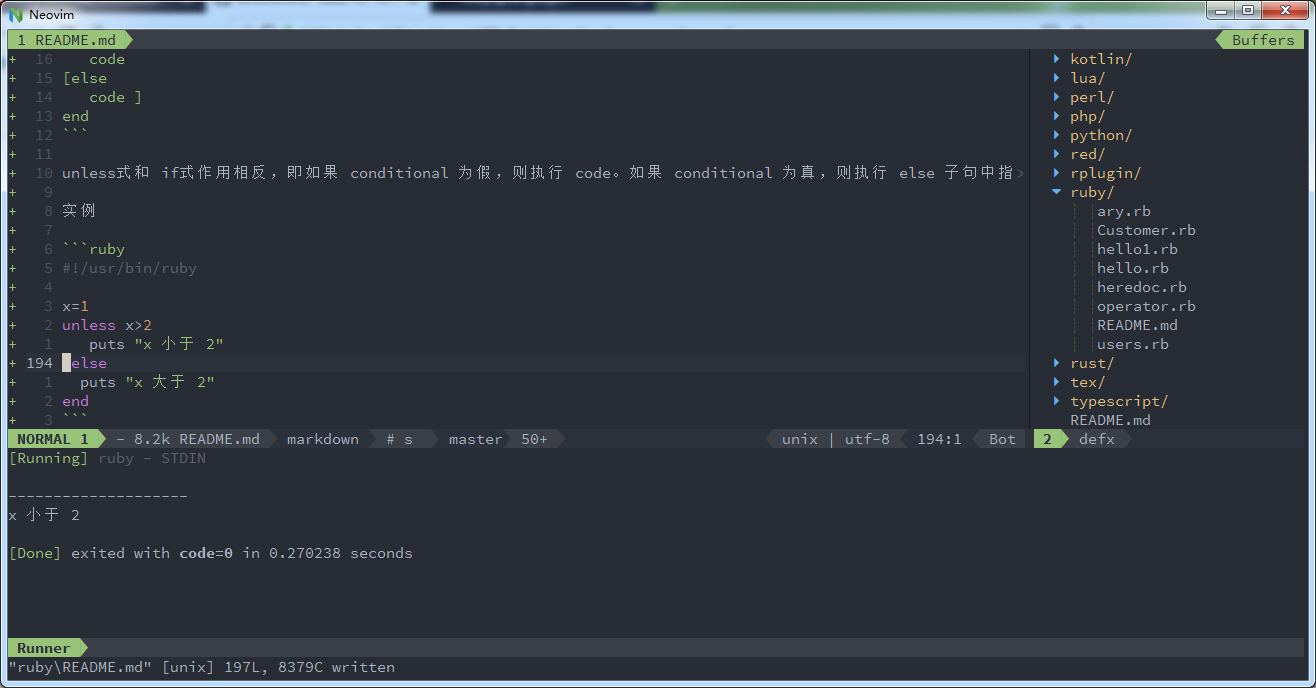## unless 修饰符

``````code unless conditional
``````

``````#!/usr/bin/ruby
# -*- coding: UTF-8 -*-

\$var =  1
print "1 -- 这一行输出\n" if \$var
print "2 -- 这一行不输出\n" unless \$var

\$var = false
print "3 -- 这一行输出\n" unless \$var
``````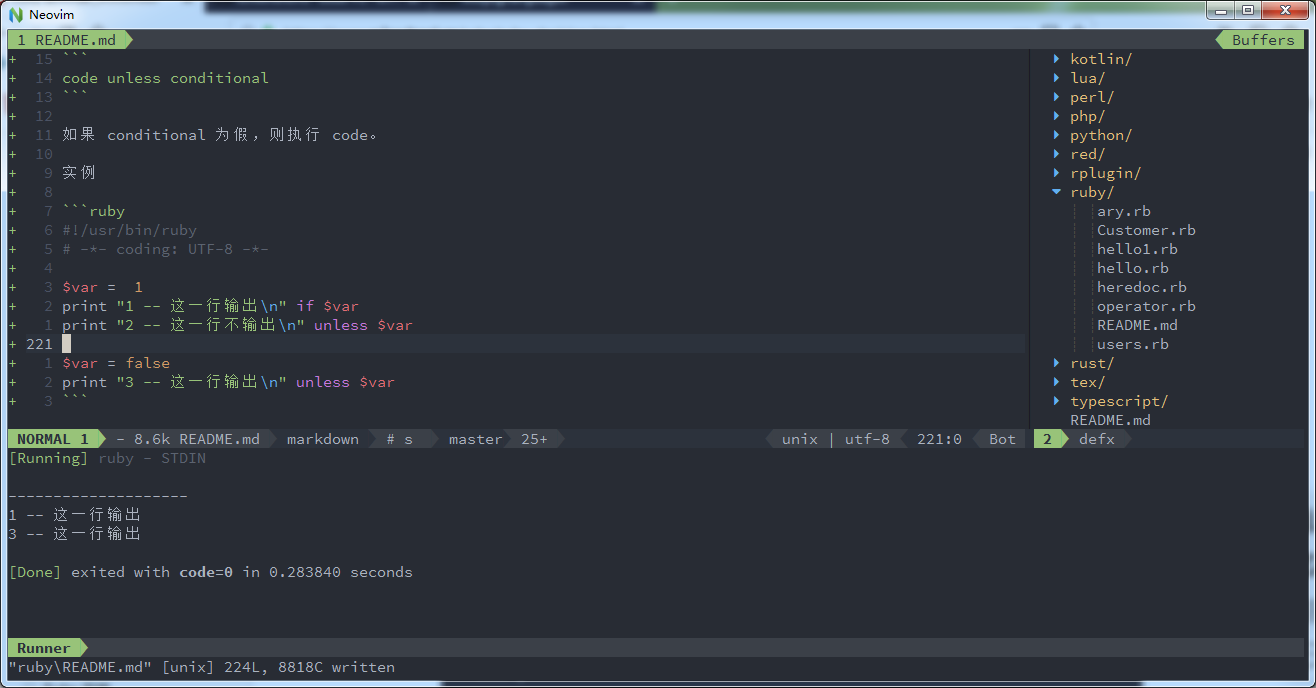## case 语句

``````case expression
[when expression [, expression ...] [then]
code ]...
[else
code ]
end
``````

case 先对一个 expression 进行匹配判断，然后根据匹配结果进行分支选择。

``````when a == 4 then a = 7 end
``````

``````case expr0
when expr1, expr2
stmt1
when expr3, expr4
stmt2
else
stmt3
end
``````

``````_tmp = expr0
if expr1 === _tmp || expr2 === _tmp
stmt1
elsif expr3 === _tmp || expr4 === _tmp
stmt2
else
stmt3
end
``````

``````#!/usr/bin/ruby
# -*- coding: UTF-8 -*-

\$age =  5
case \$age
when 0 .. 2
puts "婴儿"
when 3 .. 6
puts "小孩"
when 7 .. 12
puts "child"
when 13 .. 18
puts "少年"
else
puts "其他年龄段的"
end
``````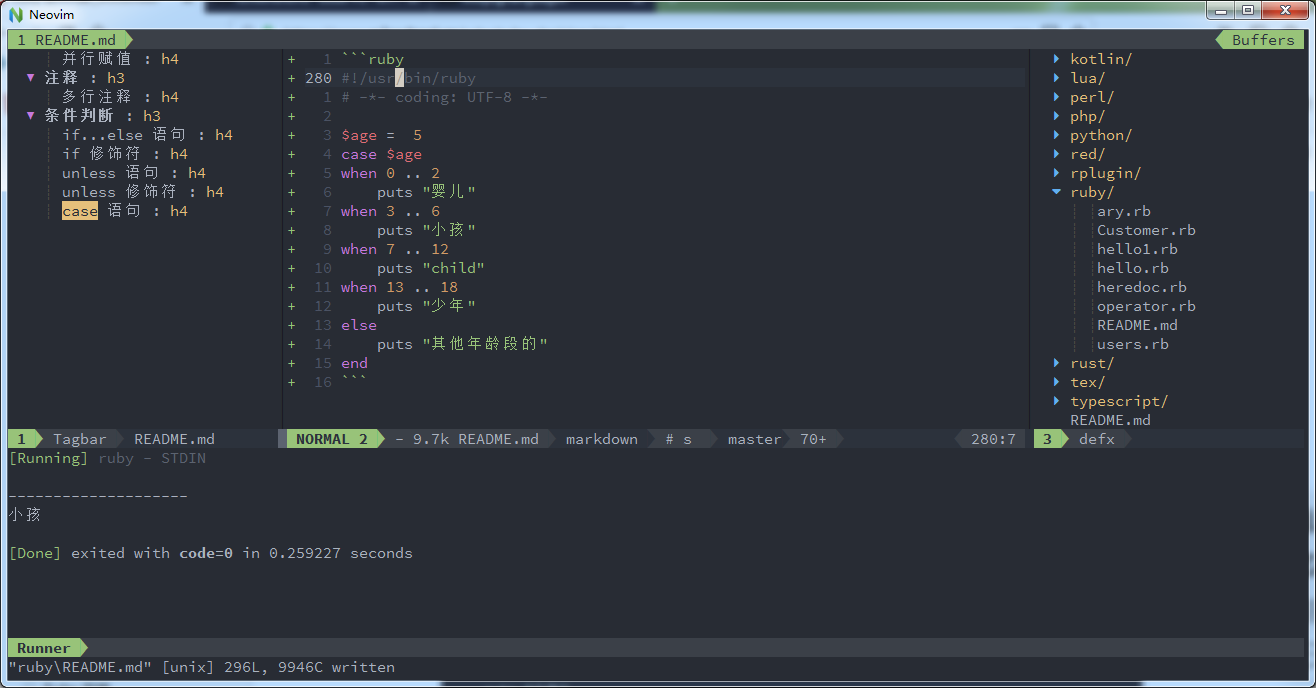Share on:

## 评论

暂无评论

使用 GitHub issue 参与评论。

目录